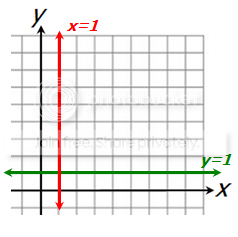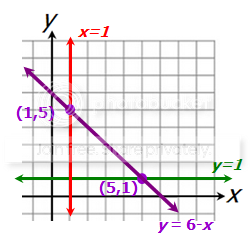GMAT Question of the Day - Daily to your Mailbox; hard ones only

 It is currently 18 Jun 2019, 06:08GMAT Club Daily Prep

Thank you for using the timer - this advanced tool can estimate your performance and suggest more practice questions. We have subscribed you to Daily Prep Questions via email.

Customized
for You

we will pick new questions that match your level based on your Timer History

Track

every week, we’ll send you an estimated GMAT score based on your performance

Practice
Pays

we will pick new questions that match your level based on your Timer History

Not interested in getting valuable practice questions and articles delivered to your email? No problem, unsubscribe here.What is the area inscribed by the lines y =1, x = 1, y = 6-x on an xy-

Author Message
TAGS:

Hide Tags

InternJoined: 10 Apr 2016
Posts: 9
What is the area inscribed by the lines y =1, x = 1, y = 6-x on an xy-  [#permalink]

Show Tags

1100:00

Difficulty:55% (hard)

Question Stats:66% (02:18) correct34% (02:15) wrongbased on 289 sessions

HideShow timer Statistics

What is the area inscribed by the lines y =1, x = 1, y = 6-x on an xy-coordinate plane?

a) 8
b) 10
c) 12
d) 14
e) 18

Originally posted by PawanRamnani1234 on 08 Sep 2016, 06:54.
Last edited by Bunuel on 08 Sep 2016, 07:05, edited 1 time in total.
Renamed the topic and edited the question.
GMAT TutorG
Status: Tutor - BrushMyQuant
Joined: 05 Apr 2011
Posts: 620
Location: India
Concentration: Finance, Marketing
Schools: XLRI (A)
GMAT 1: 700 Q51 V31GPA: 3
WE: Information Technology (Computer Software)
Re: area inscribed by the lines.  [#permalink]

Show Tags

2
Top Contributor
Check the attached image

We are supposed to find the area of the figure enclosed by the lines x=1, y=1 and y=6-x which is nothing but Area of Triangle ABC.
AB = 4 (distance between (1,1) and (5,1))
AC = 4 (distance between (1,1) and (1,5))

Area of Triangle ABC which is right angled at A = (1/2) * AB * AC = (1/2)*4*4 = 8

Hope it helps!

PawanRamnani1234 wrote:
What is the area inscribed by the lines y =1, x = 1, y = 6-x on an xy-coordinate plane?

a) 8
b) 10
c) 12
d) 14
e) 18

Looking for appropriate solution for above problem.

Attachments

File comment: Figureimage.jpg [ 61.4 KiB | Viewed 3718 times ]

_________________
CEOV
Joined: 12 Sep 2015
Posts: 3782
Re: What is the area inscribed by the lines y =1, x = 1, y = 6-x on an xy-  [#permalink]

Show Tags

1
Top Contributor
PawanRamnani1234 wrote:
What is the area inscribed by the lines y =1, x = 1, y = 6-x on an xy-coordinate plane?

a) 8
b) 10
c) 12
d) 14
e) 18

First, let's graph the lines y = 1 and x = 1At this point, we need to find the points where the line y = 6-x INTERSECTS the other two lines.

For the vertical line, we know that x = 1, so we'll PLUG x = 1 into the equation y = 6-x to get y = 6-1 = 5
Perfect, when x = 1, y = 5, so one point of intersection is (1,5)

For the horizontal line, we know that y = 1, so we'll PLUG y = 1 into the equation y = 6-x to get 1 = 6-x. Solve to get: x = 5
So, when y = 1, x = 5, so one point of intersection is (5,1)

Now add these points to our graph and sketch the line y = 5-xAt this point, we can see that we have the following triangle.The base has length 4 and the height is 4
Area = (1/2)(base)(height)
= (1/2)(4)(4)
= 8

RELATED VIDEOS

_________________
Math ExpertV
Joined: 02 Aug 2009
Posts: 7757
Re: What is the area inscribed by the lines y =1, x = 1, y = 6-x on an xy-  [#permalink]

Show Tags

PawanRamnani1234 wrote:
What is the area inscribed by the lines y =1, x = 1, y = 6-x on an xy-coordinate plane?

a) 8
b) 10
c) 12
d) 14
e) 18

Hi,

The sketches have been made above, but few points..
1) Three equation,so three lines and therefore a triangle..two sides x=1and y=1 make a right angle so we are talking of a RIGHT angle triangle..
2) the third line will intersect these two lines ..
On line x=1, when x is 1 in y=6-x, y=6-1=5.. similarly on line y=1at x=5..
3) therefore the length of each side is 5-1=4..
Area =1/2*4*4=8..
A
_________________
DirectorS
Joined: 12 Nov 2016
Posts: 715
Location: United States
Schools: Yale '18
GMAT 1: 650 Q43 V37GRE 1: Q157 V158GPA: 2.66
Re: What is the area inscribed by the lines y =1, x = 1, y = 6-x on an xy-  [#permalink]

Show Tags

PawanRamnani1234 wrote:
What is the area inscribed by the lines y =1, x = 1, y = 6-x on an xy-coordinate plane?

a) 8
b) 10
c) 12
d) 14
e) 18

There's no need to actually diagram this problem- simply not much time on the GMAT; however, it might be ok in the beginning. Anyways, the concept behind this question is simply testing your ability to plug in the given y and x values in order to find the two other vertices of the figure- which if you do diagram you'll see is an isosceles equilateral triangle.

1= 6-x
-5=-x
5=x (we're not done yet though)

y=6-(x)
y=6- 1
y= 5 ( we are done yet either)

x and y intersect at (1,1) so actually each length of this triangle would be 4= 4 spots upwards from (1,1) actually gives us (1,5) - the vertice of the triangle derived from plugging in our x value; And 4 spots to the right of (1,1) gives us (5,1) the vertice of the triangle derived from plugging in the y value. And because this triangle is a right triangle (the intersection of two perfectly straight lines, lines with a slope of 0, is 90 degrees and the lines x and y are also perpendicular) the area can be calculated simply by

base1 x base 2 / 2 = area
(4) x (4)/2 =
16/2= 8

Thus

"A"
ManagerB
Joined: 11 Feb 2017
Posts: 187
Re: What is the area inscribed by the lines y =1, x = 1, y = 6-x on an xy-  [#permalink]

Show Tags

PawanRamnani1234 wrote:
What is the area inscribed by the lines y =1, x = 1, y = 6-x on an xy-coordinate plane?

a) 8
b) 10
c) 12
d) 14
e) 18

Let me ask u guys one question

btw I understood this question but here is a doubt

When we are asked to make a graph from a certain equation then we put x=0 and calculate y THEN put y=0 and calculate x and then we plot it

BUT WHY HERE WE TOOK VALUE OF x=1 from the first equation and placed in 3rd equation????
WHY we did that for y as well?

Non-Human UserJoined: 09 Sep 2013
Posts: 11384
Re: What is the area inscribed by the lines y =1, x = 1, y = 6-x on an xy-  [#permalink]

Show Tags

Hello from the GMAT Club BumpBot!

Thanks to another GMAT Club member, I have just discovered this valuable topic, yet it had no discussion for over a year. I am now bumping it up - doing my job. I think you may find it valuable (esp those replies with Kudos).

Want to see all other topics I dig out? Follow me (click follow button on profile). You will receive a summary of all topics I bump in your profile area as well as via email.
_________________Re: What is the area inscribed by the lines y =1, x = 1, y = 6-x on an xy-   [#permalink] 21 Sep 2018, 22:49
Display posts from previous: Sort by

What is the area inscribed by the lines y =1, x = 1, y = 6-x on an xy-